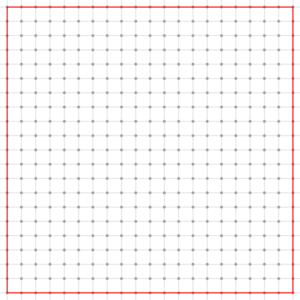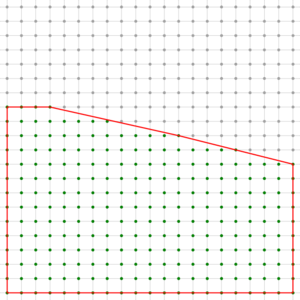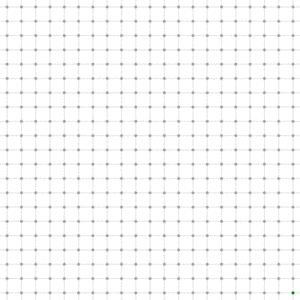DGtal  1.3.beta
Lattice polytopes in the digital plane ZxZ (convex polygons with vertices at integer coordinates)
Author(s) of this documentation:
Jacques-Olivier Lachaud

Part of the Arithmetic package.

This module gathers classes and functions to represent lattice polytopes in 2D (otherwise saide, convex polygons with vertices at integer coordinates) and digital half-spaces. The main part of the module is the class LatticePolytope2D, which represents these polytopes. This module is a possible solution for solving integer linear programming in the plane. It is thus the basis for the COBA algorithm for recognizing digital planes (see COBANaivePlaneComputer).

# Creating a lattice polytope in Z2

The class LatticePolytope2D represents an arbitary convex polygon in the 2D plane whose vertices have integer coordinates. It is parameterized by a digital space TSpace (a model of CSpace with dimension 2) and by the container class TSequence for storing vertices (a model of [boost::Sequence http://www.sgi.com/tech/stl/Sequence.html] over TSpace::Point, default is std::list<TSpace::Point>). The class LatticePolytope2D contains as a data member some TSequence instance and you may use all the standard methods of sequences. All the useful data of a LatticePolytope2D are in the TSequence data member.

The class LatticePolytope2D is a model of boost::Sequence, but also of boost::DefaultConstructible, boost::CopyConstructible, boost::Assignable. This class is also a model of CDrawableWithBoard2D. You may therefore display it on a Board2D object.

Since a sequence is ordered, its order defines the order of vertices along the polygon, i.e. any vertex in the sequence forms an edge with the previous vertex and another edge with the next vertex. Note that the vertices must follow the clockwise ordering, i.e. the inside of the polygon is to the right-hand side when moving along the polygon. The following example defines a triangle in the standard limited integer planes Z2i::Z2 (integer coordinates are int32_t):

#include "DGtal/arithmetic/LatticePolytope2D.h"
using namespace Z2i;
...
typedef LatticePolytope2D<Z2> CIP;
CIP triangle;
triangle.push_front( Point( 0, 0 ) );
triangle.push_front( Point( 0, 7 ) );
triangle.push_front( Point( 10, 0 ) );
Note
This class contains a mutable object IntegerComputer as well as several other mutable members to perform computations efficiently (see dgtal_integer_computations). However these private data members are not copied nor assigned. They exists so as to avoid memory managements when using big integers.

You may use any insertion/deletion methods of a sequence (insert, erase, push_back, push_front, etc). Be careful however, in order that the object has a correct behaviour in more elaborate methods like LatticePolytope2D::cut() or LatticePolytope2D::findCut, you must enforce that inserted or deleted vertices leave the polytope convex.

# Displaying 2D lattice polytopes

A LatticePolytope2D is a model of CDrawableWithBoard2D. Therefore it is displayable on a Board2D with the operator <<. The following example displays a square in red over its domain.

CIP cip;
cip.push_front( Point( -10, -10 ) );
cip.push_front( Point( -10, 10 ) );
cip.push_front( Point( 10, 10 ) );
cip.push_front( Point( 10, -10 ) );
Domain domain = cip.boundingBoxDomain();
Board2D board;
board << domain
<< CustomStyle( cip.className(),
new CustomColors( Color::Red, Color::None ) )
<< cip;
board.saveEPS( "lower-integer-convex-hull.eps" );
board.clear();

You may choose colors with the usual CustomStyle / CustomColors mechanism. Furthermore, choosing Color::None for filling colors allows to draw only the boundary of the polygon.Display of a LatticePolytope2D object in red (a square of side 20)

# Converting a 2D lattice polytope into a digital set

It is sometimes useful to enumerate all the integer points lying within the polygon bounds. The method LatticePolytope2D::getIncludedDigitalPoints() is templated by the type TDigitalSet, which must be a model of CDigitalSet. It modifies the digital set given in parameter so as to holds these points. For now, the complexity is not optimal. If D is the size of the domain of TDigitalSet, N the number of vertices of the polygon, then the complexity is upper bounded by O(ND log(D)) (if TDigitalSet is DigitalSetBySTLSet).

// cip is some lattice polytope
Domain domain = cip.boundingBoxDomain();
cip.getIncludedDigitalPoints( aSet );
// aSet contains the digital points inside or on the boundary of the polygon.
board << aSet; // displays them.

# Basic services: vertices, centroid, area

Since a LatticePolytope2D is a sequence, you may iterate over the vertices with the usual LatticePolytope2D::begin()and LatticePolytope2D::end(), with two versions of iterators (Iterator and ConstIterator). To know if the polygon has no vertices, it is faster to use LatticePolytope2D::empty(). Otherwise you may obtain the following information:

A LatticePolytope2D P may have zero area (in this case it has at most two vertices). Thanks to Pick formula, you can also get the exact number b of points on the boundary and the exact number i of points in the interior of the polygon: $$A(P) = i + b/2 - 1$$.

std::cout << "Number of vertices = " << cip.size() << std::endl;
std::cout << "Area = " << (((double)cip.twiceArea())/2.0) << std::endl;
std::cout << "Number of interior points = " << cip.numberInteriorPoints() << std::endl;
std::cout << "Number of boundary points = " << cip.numberBoundaryPoints() << std::endl;

# Polygon edges define half-planes

A lattice polytope polygon may be defined by a list of vertices (with some properties) but also as the intersection of half-planes. For instance, if $$(v_i)_{i=0..n-1}$$ are the vertices of the polytope, then the polytope is also the intersection of the half-planes which contains the polytope centroid and whose boundary contains edges $$(v_i,v_{i+1})$$.

You may use the following methods:

# Cutting the polygon by half-planes

You may also update a LatticePolytope2D by intersecting it with half-planes, i.e. linear constraints of the form ax+by <= c. The polygon is updated so that all its vertices have integer coordinates and the constraints are fulfilled. This means for instance that a set of linear constraints may have a non-empty interior (in the Euclidean space sense), but the resulting polygon may be empty or have an empty interior. However, it is guaranteed that all integer solutions are kept.

This snippet is taken from example lower-integer-convex-hull.cpp. It cuts the polygon by calling LatticePolytope2D::cut. An half-plane is specified beforehands by instantiating a LatticePolytope2D::HalfSpace. Note that it is a redefinition of the class ClosedIntegerHalfPlane.

HalfSpace hs( Vector( a, b ), c );
cip.cut( hs );
DigitalSet aSet( domain );
board << domain
<< CustomStyle( aSet.className(),
new CustomColors( Color::Green, Color::Green ) )
<< SetMode( Point().className(), "Grid" )
<< aSet
<< CustomStyle( cip.className(),
new CustomColors( Color::Red, Color::None ) )
<< cip;
board.saveEPS( "lower-integer-convex-hull-cut.eps" );Square of side 20 cut by the half-plane 3x+13y <= 19

This shows the successive polygons obtained by cutting a square with constraints -5x+8y <= c.Square cut by the half space -5x+8y <= c, for c from -130 to 130
DGtal::Z2i::Point
Space::Point Point
Definition: StdDefs.h:95
DGtal::Color::Green
static const Color Green
Definition: Color.h:417
DGtal::Shapes::makeSetFromPointPredicate
static void makeSetFromPointPredicate(DigitalSet &aSet, const PointPredicate &aPP)
DGtal::Color::Red
static const Color Red
Definition: Color.h:416
Domain
HyperRectDomain< Space > Domain
Definition: testSimpleRandomAccessRangeFromPoint.cpp:44
DGtal::LatticePolytope2D::HalfSpace
ClosedIntegerHalfPlane< Space > HalfSpace
Definition: LatticePolytope2D.h:98
Vector
FreemanChain< int >::Vector Vector
Definition: testCombinDSS.cpp:60
domain
Domain domain
Definition: testProjection.cpp:88
DGtal::Color::None
static const Color None
Definition: Color.h:412
Point
MyPointD Point
Definition: testClone2.cpp:383
DGtal::DigitalSetByAssociativeContainer
Aim: A wrapper class around a STL associative container for storing sets of digital points within som...
Definition: DigitalSetByAssociativeContainer.h:89